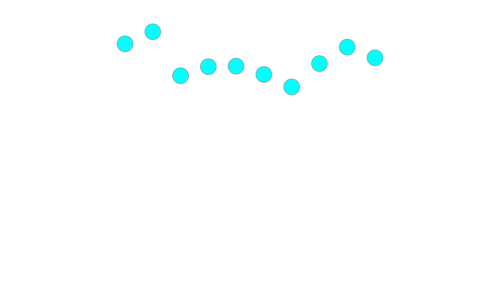# PyGfx picking example¶

Note that this example depends on pygfx (pip install -U pygfx).

An example demonstrating pickable points.

:

import numpy as np
import pygfx as gfx
from wgpu.gui.jupyter import WgpuCanvas

class PickingWgpuCanvas(WgpuCanvas):
def handle_event(self, event):
super().handle_event(event)
# Get a dict with info about the clicked location
if event["event_type"] == "pointer_down":
xy = event["x"], event["y"]
info = renderer.get_pick_info(xy)
wobject = info["world_object"]
# If a point was clicked ..
if wobject and "vertex_index" in info:
i = int(round(info["vertex_index"]))
geometry.positions.data[i, 1] *= -1
geometry.positions.update_range(i)
canvas.request_draw()

canvas = PickingWgpuCanvas()
renderer = gfx.renderers.WgpuRenderer(canvas)
scene = gfx.Scene()

xx = np.linspace(-50, 50, 10)
yy = np.random.uniform(20, 50, 10)
geometry = gfx.Geometry(positions=[(x, y, 0) for x, y in zip(xx, yy)])
if True:  # Set to False to try this for a line
ob = gfx.Points(geometry, gfx.PointsMaterial(color=(0, 1, 1, 1), size=16))
else:
ob = gfx.Line(geometry, gfx.LineMaterial(color=(0, 1, 1, 1), thickness=10))

camera = gfx.OrthographicCamera(120, 120)

canvas.request_draw(lambda: renderer.render(scene, camera))
canvas

Forcing backend: Vulkan (4)initial snapshot
:

[ ]: